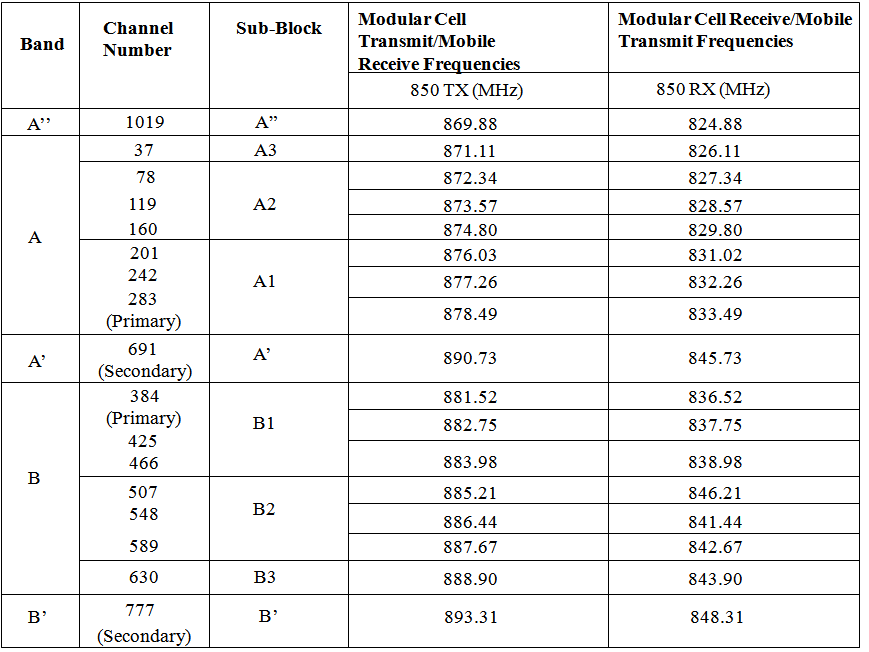TELETOPIX.ORG

Telecom Techniques Guide

CDMA

# CDMA Channel Frequencies & CalculationThe 850MHz CDMA band is most popularly used all over the world.This band as mentioned in the previous slide works between
824-849MHz Used for the Reverse link communication
869-894MHz Used for the Forward link communication
The CDMA band is divided into sub bands as shown above.The Total Band of  25MHz is divided into small channels of 30KHz each.An actual CDMA carrier will be using a multiple of the 30KHz channels.
That means for  an actually utilized bandwidth of 1.23MHz we will need 41X30KHz channels.
The Following equation gives the relationship between the channel numbers and the actual frequency.
Reverse Link Frequency = (825 + N0.03)MHz
Forward Link Frequency = (870 + N0.03)MHz
Where N = CDMA channel number